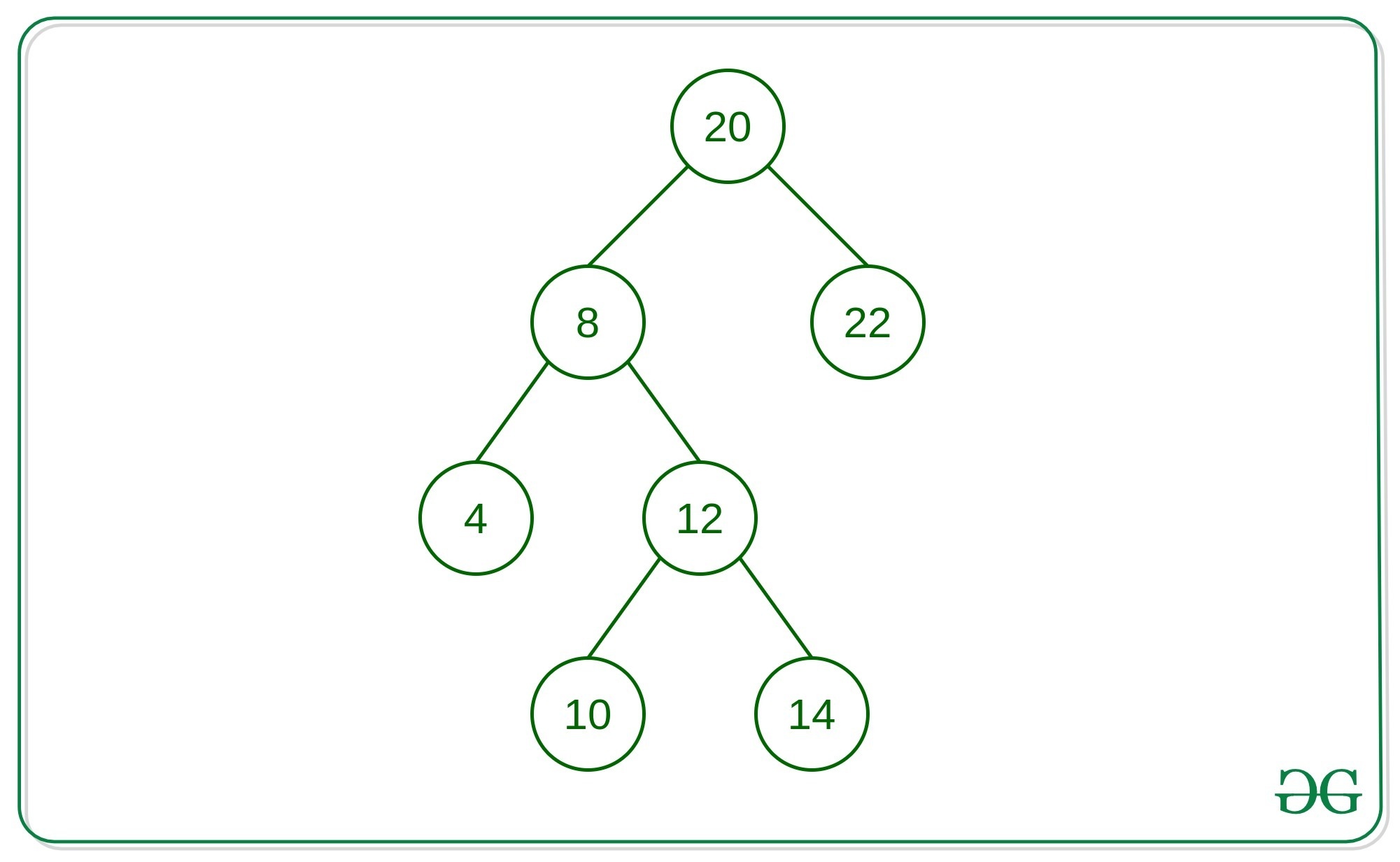# Print all nodes at distance K from given node: Iterative Approach

Given a binary tree, a target node and an integer K, the task is to find all the nodes that are at distance K from the given target node.Consider the above-given Tree, For the target node 12.
Input: K = 1
Output: 8 10 14

Input: K = 2
Output: 4 20

Input: K = 3
output: 22

## Recommended: Please try your approach on {IDE} first, before moving on to the solution.

Approach:
There are generally two cases for the nodes at a distance of K:

1. Node at a distance K is a child node of the target node.
2. Node at a distance K is the ancestor of the target node.

The idea is to store the parent node of every node in a hash-map with the help of the Level-order traversal on the tree. Then, Simply Traverse the nodes from the Target node using Breadth-First Search on the left-child, right-child, and the parent node. At any instant when the distance of a node the from the target node is equal to K then print all the nodes of the queue.

Below is the implementation of the above approach:

## C++

 `// C++ implementation to print all ` `// the nodes from the given target  ` `// node iterative approach ` ` `  `#include ` ` `  `using` `namespace` `std; ` ` `  `// Structure of the Node ` `struct` `Node { ` `    ``int` `val; ` `    ``Node *left, *right; ` `}; ` ` `  `// Map to store the parent  ` `// node of every node of  ` `// the given Binary Tree ` `unordered_map um; ` ` `  `// Functiom to store all nodes ` `// parent in unordered_map ` `void` `storeParent(Node* root) ` `{ ` ` `  `    ``// Make a queue to do level-order ` `    ``// Traversal and store parent ` `    ``// of each node in unordered map ` `    ``queue q; ` `    ``q.push(root); ` `     `  `    ``// Loop to iterate until the  ` `    ``// queue is not empty ` `    ``while` `(!q.empty()) { ` `        ``Node* p = q.front(); ` `        ``q.pop(); ` `         `  `        ``// Condition if the node is a  ` `        ``/// root node that storing its  ` `        ``// parent as NULL ` `        ``if` `(p == root) { ` `            ``um[p] = NULL; ` `        ``} ` `         `  `        ``// if left child exist of  ` `        ``// popped out node then store  ` `        ``// parent as value and node as key ` `        ``if` `(p->left) { ` `            ``um[p->left] = p; ` `            ``q.push(p->left); ` `        ``} ` `        ``if` `(p->right) { ` `            ``um[p->right] = p; ` `            ``q.push(p->right); ` `        ``} ` `    ``} ` `} ` ` `  `// Function to find the nodes  ` `// at distance K from give node ` `void` `nodeAtDistK(Node* root,  ` `           ``Node* target, ``int` `k) ` `{ ` `    ``// Keep track of each node  ` `    ``// which are visited so that  ` `    ``// while doing BFS we will  ` `    ``// not traverse it again ` `    ``unordered_set s; ` `    ``int` `dist = 0; ` `    ``queue q; ` `    ``q.push(target); ` `    ``s.insert(target); ` `     `  `    ``// Loop to iterate over the nodes ` `    ``// until the queue is not empty ` `    ``while` `(!q.empty()) { ` ` `  `        ``// if distance is equal to K ` `        ``// then we found a node in tree ` `        ``// which is distance K ` `        ``if` `(dist == k) { ` `            ``while` `(!q.empty()) { ` `                ``cout << q.front()->val << ``" "``; ` `                ``q.pop(); ` `            ``} ` `        ``} ` ` `  `        ``// BFS on node's left,  ` `        ``// right and parent node ` `        ``int` `size = q.size(); ` `        ``for` `(``int` `i = 0; i < size; i++) { ` `            ``Node* p = q.front(); ` `            ``q.pop(); ` ` `  `            ``// if the left of node is not  ` `            ``// visited yet then push it in  ` `            ``// queue and insert it in set as well ` `            ``if` `(p->left &&  ` `                ``s.find(p->left) == s.end()) { ` `                ``q.push(p->left); ` `                ``s.insert(p->left); ` `            ``} ` ` `  `            ``// if the right of node is not visited ` `            ``// yet then push it in queue  ` `            ``// and insert it in set as well ` `            ``if` `(p->right &&  ` `                ``s.find(p->right) == s.end()) { ` `                ``q.push(p->right); ` `                ``s.insert(p->right); ` `            ``} ` ` `  `            ``// if the parent of node is not visited  ` `            ``// yet then push it in queue and  ` `            ``// insert it in set as well ` `            ``if` `(um[p] && s.find(um[p]) == s.end()) { ` `                ``q.push(um[p]); ` `                ``s.insert(um[p]); ` `            ``} ` `        ``} ` `        ``dist++; ` `    ``} ` `} ` ` `  `// Function to create a newnode ` `Node* newnode(``int` `val) ` `{ ` `    ``Node* temp = ``new` `Node; ` `    ``temp->val = val; ` `    ``temp->left = temp->right = NULL; ` `    ``return` `temp; ` `} ` ` `  `// Driver Code ` `int` `main() ` `{ ` `    ``Node* root = newnode(20); ` `    ``root->left = newnode(8); ` `    ``root->right = newnode(22); ` `    ``root->right->left = newnode(5); ` `    ``root->right->right = newnode(8); ` `    ``root->left->left = newnode(4); ` `    ``root->left->left->left = newnode(25); ` `    ``root->left->right = newnode(12); ` `    ``root->left->right->left =  ` `                   ``newnode(10); ` `    ``root->left->right->left->left =  ` `                   ``newnode(15); ` `    ``root->left->right->left->right =  ` `                   ``newnode(18); ` `    ``root->left->right->left->right->right =  ` `                   ``newnode(23); ` `    ``root->left->right->right =  ` `                   ``newnode(14); ` `    ``Node* target = root->left->right; ` `    ``storeParent(root); ` `    ``nodeAtDistK(root, target, 3); ` `    ``return` `0; ` `} `

Don’t stop now and take your learning to the next level. Learn all the important concepts of Data Structures and Algorithms with the help of the most trusted course: DSA Self Paced. Become industry ready at a student-friendly price.

My Personal Notes arrow_drop_upCheck out this Author's contributed articles.

If you like GeeksforGeeks and would like to contribute, you can also write an article using contribute.geeksforgeeks.org or mail your article to contribute@geeksforgeeks.org. See your article appearing on the GeeksforGeeks main page and help other Geeks.

Please Improve this article if you find anything incorrect by clicking on the "Improve Article" button below.

Article Tags :
Practice Tags :

6

Please write to us at contribute@geeksforgeeks.org to report any issue with the above content.